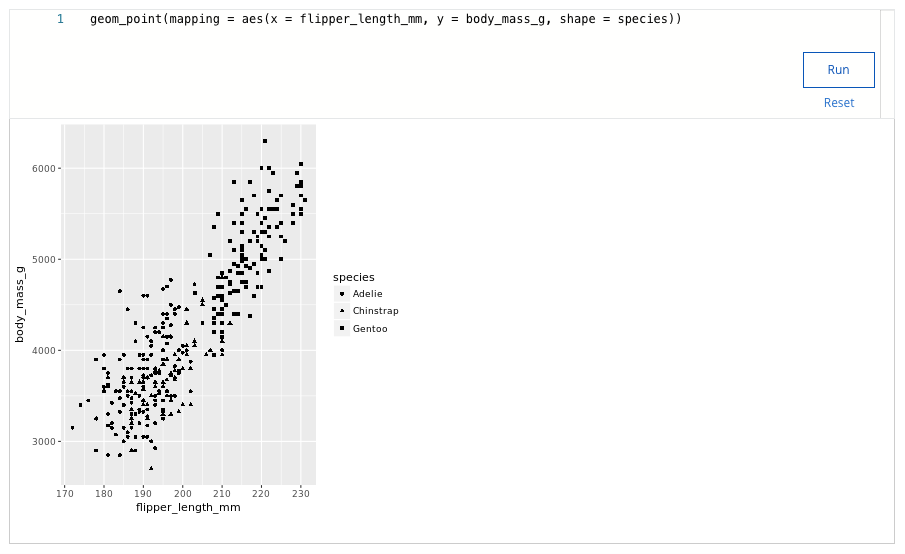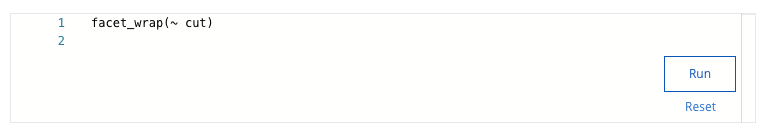## Week 4 – More about visualizations, aesthetics, and annotations – Shuffle Q/A 3

#### 37. You are working with the penguins dataset. You create a scatterplot with the following code chunk: ggplot(data = penguins) + geom_point(mapping = aes(x = flipper_length_mm, y = body_mass_g))You want to highlight the different years of data collection on your plot. Add a code chunk to the second line of code to map the aesthetic alpha to the variable island.NOTE: the three dots (...) indicate where to add the code chunk. You may need to scroll in order to find the dots.#### What islands does your visualization display?

• Biscoe, Dream, Torgersen
• Cebu, Borneo, Torgersen
• Cebu, Java, Hispaniola
• Biscoe, Java, Buton

#### 38. What function creates a scatterplot and then adds a small amount of random noise to each point in the plot to make the points easier to find?

• The geom_smooth() function
• The geom_jitter() function
• The geom_point() function
• The geom_bar() function

• annotate()
• labs()
• facet()
• text()

#### 40. You are working with the penguins dataset. You create a scatterplot with the following lines of code: ggplot(data = penguins) + geom_point(mapping = aes(x = flipper_length_mm, y = body_mass_g)) + What code chunk do you add to the third line to save your plot as a pdf file with “penguins” as the file name?

• ggsave(penguins.pdf)
• ggsave(“pdf.penguins”)
• ggsave(=penguins)
• ggsave(“penguins.pdf”)

#### 41. A data analyst creates a plot using the following code chunk: ggplot(data = penguins) + geom_point(mapping = aes(x = flipper_length_mm, y = body_mass_g))Which of the following represents an aesthetic attribute in the code chunk? Select all that apply.

• flipper_length_mm
• body_mass_g
• y
• x

• text
• note
• title
• annotate

• HTML
• Image
• Slideshow
• PDF

#### 44. Fill in the blank: In ggplot2, you use the _____ to add layers to your plot.

• The plus sign (+)
• The pipe operator (%>%)
• The equals sign (=)
• The ampersand symbol (&)

#### 45. A data analyst is working with the penguins data. The analyst creates a scatterplot with the following code: ggplot(data = penguins) + geom_point(mapping = aes(x = flipper_length_mm, y = body_mass_g, alpha = species))What does the alpha aesthetic do to the appearance of the points on the plot?

• Makes the points on the plot larger
• Makes some points on the plot more transparent
• Makes the points on the plot smaller
• Makes the points on the plot more colorful

#### 46. You are working with the diamonds dataset. You create a bar chart with the following code:ggplot(data = diamonds) + geom_bar(mapping = aes(x = color, fill = cut)) +You want to use the facet_wrap() function to display subsets of your data. Add the code chunk that lets you facet your plot based on the variable cut.• 3
• 4
• 5
• 6